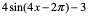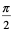# Problem of the Day March 25, 2020

Mar 25, 2020March 25, 2020

What it the ratio of books to boxes when you have 42 books and 7 boxes?

What is the rate? What is the difference between a rate and a ratio? Give an example of when you might use a rate?

Solve the following equations for x:

2x + 5 = 23

4x - 7 = 21

3x = 72

Find a set of 4 points that solve the following linear equation:

-3x + 4y = -2

Algebra:

Solve the following system of equations:

2x - 5y = 6

4x + 10y = 20

Geometry:

Describe the following geometric shapes. How are they alike? How are they different?  parallelogram, rhombus, square, rectangle

Algebra 2:

Solve the following absolute value equations:

2| 3x - 6 | = 48

| 2x + 5 | = - 23

| 5x + 7 | -6 = 41

Trigonometry:

Give the amplitude, maximum and minimum values (range), period, vertical and horizontal shift of the following sin function:Pre-Calculus

Find the sum, difference, product and quotient of the following two functions: f(x) = 2x - 3  and g(x) = 10x2 -11x - 6

Grade 6: ratio 42 : 7  rate 6 : 1  A ratio is a comparison between 2 numbers whose units don’t have to be the same ( for example pitches : game, apples : oranges) Remember ratios can also be written like fractions

pitches    ,     apples         A rate is a ratio of measurements that have two

game             oranges

different units and is typically written with a denominator of 1.  You would find it helpful to use a rate when comparing boxes of cereal that are difference sizes to see which is the better buy or which car can travel further on a gallon of gas.

Grade 7:  2x + 5 = 23  x = 9     4x - 7 = 21  x = 7    3x = 72  x = 24

Grade 8:  many answers some would be ( 2 , 1 )    ( 6 , 4 )    ( -2, -2 )

Algebra:  x = 4   y = 2/5

Geometry:  All 4 shapes have opposite sides parallel. Square and rhombus have all 4 sides congruent. Rectangle and parallelogram have opposites side congruent. Square and rectangle all angles are 90 degrees. Rhombus and parallelogram have opposite angles congruent and consecutive angles supplementary. Square and rectangle have diagonals that are congruent. All four have diagonals that bisect each other.

Algebra 2:  2 |3x - 6 | = 48  x = 10 , -6

| 2x + 5 | = -23  no solution

| 5x + 7 | - 6 = 41  x = 8 , -54/5

Trigonometry:  amplitude 4   range [ -7, 1]  period ishorizontal shift is rightvertical shift is down 3

Pre-Calculus:  sum  10x2 -9x -  9   difference  10x2 -13x + 3

product  20x3 - 52x2 +21x + 18

quotient  5x + 2